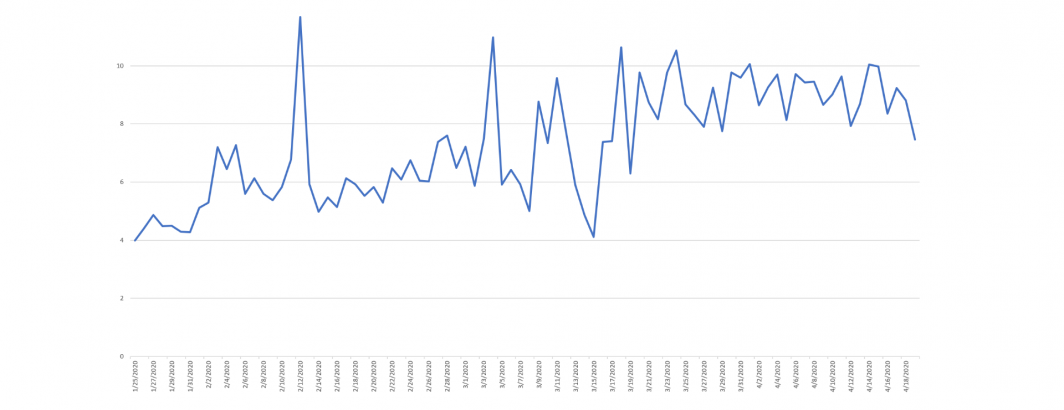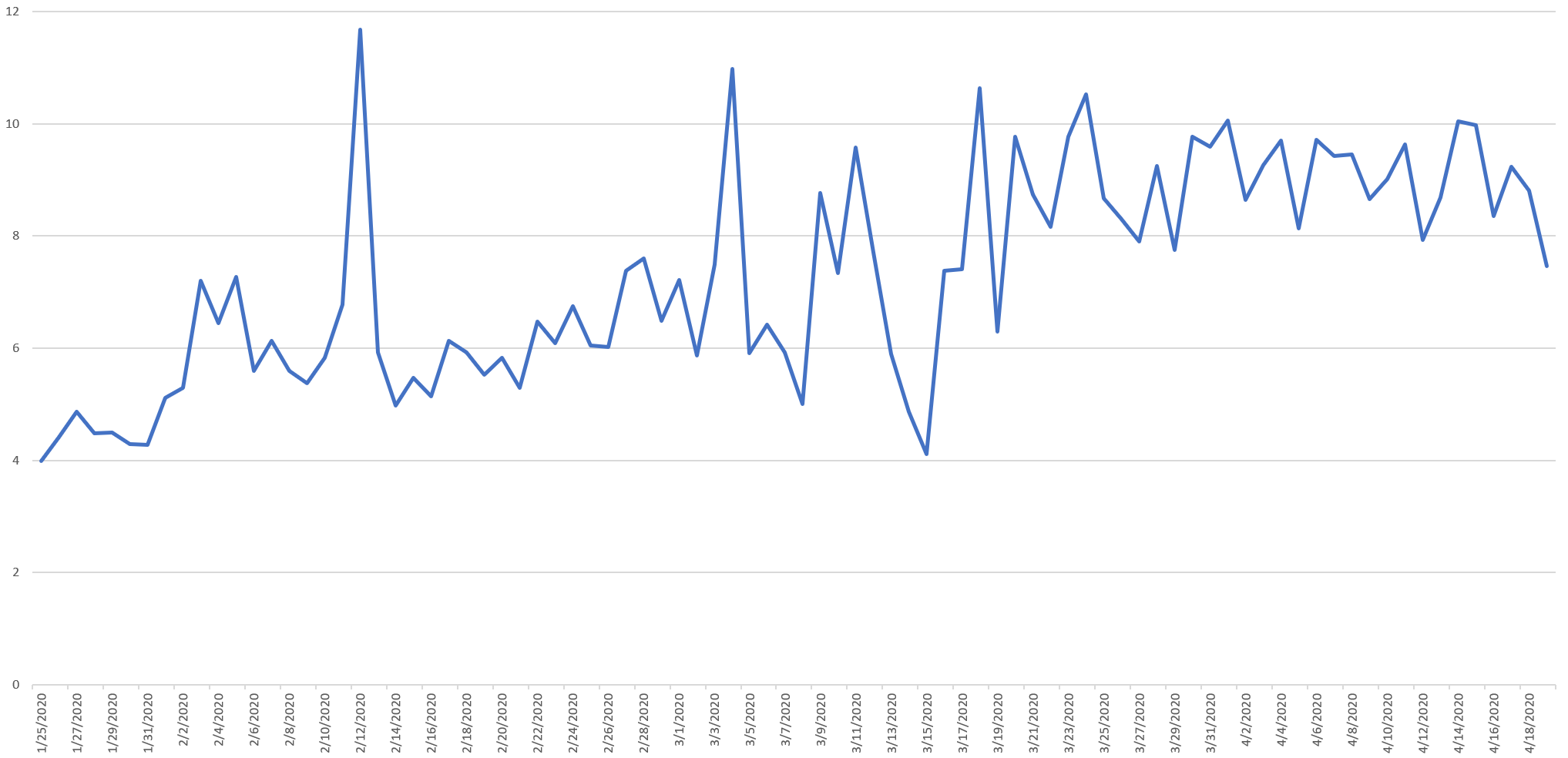# Increasing Portion Of Onscreen Text On CNN Is NumbersIn the midst of a pandemic, it might make sense for an increasing percentage of onscreen text to be numbers, reflecting a greater reliance on statistics and counts. The timeline below confirms this, reporting the total percentage of non-punctuation text each day on CNN since January 25th that was a number. In more precise terms, for each day, it sums up the total number of number characters and divides it by the total number of combined letter and number characters, showing a rampup starting around Feb. 15 and stabilizing around March 18th at its current level.## TECHNICAL DETAILS

Constructing the timeline above took just a single SQL statement.

`SELECT DATE(date) day, sum(length(REGEXP_REPLACE(OCRText,r'[^A-Za-z0-9]',''))) tot, sum(length(REGEXP_REPLACE(OCRText,r'[^0-9]',''))) totnum FROM `gdelt-bq.gdeltv2.vgegv2_iatv` WHERE DATE(date) >= "2020-01-25" and station='CNN' group by day order by day asc`